# Problem-4 (Materials and labor variances; computations from incomplete data)

Sapna company produces a single product known as product X. The company has set the following direct materials and direct labor standards for product X: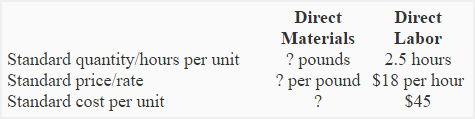During the month of August, the company produced 1,400 units of product X. A total of 6,000 pounds of direct materials was purchased at a total cost of \$33,000. The total direct labor cost for the month was \$57,000. All materials purchased during the month was used in production. There was no direct materials inventory on hand at the start and at the end of August.

Someone from the company’s management has computed the following three variances:

Required:

1. Compute the standard price per pound of materials.
2. Compute the standard quantity of materials allowed for actual production.
3. Compute the standard quantity of direct materials allowed for one unit of product X.
4. Compute the actual direct labor cost per hour for month of August.
5. Compute the direct labor rate variance. Also indicate whether it is favorable or unfavorable.

## Solution:

### (1) Standard price per pound of materials:

This requirement can be completed in three steps – computation of materials price variance, computation of actual quantity purchased at standard price and computation of standard price per pound of materials (the actual answer to requirement 1).

Step 1: Computation of materials price variance: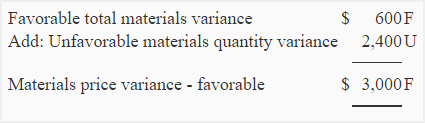Step 2: Computation of actual quantity purchased at standard price: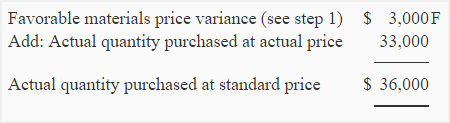Step 3: Computation of standard price per pound of materials:

Standard price per pound of materials = Actual quantity purchased at standard price/Actual quantity purchased

\$36,000/6,000 pounds

= \$6 per pound

### (2) Standard quantity of materials allowed for actual production: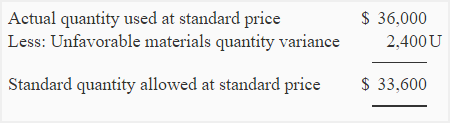Standard quantity allowed for actual production = Standard quantity allowed at standard price/Standard price per pound

= \$33,600/\$6*

= 5,600 pounds

*See answer to requirement 1

### (3) Standard quantity of materials allowed per unit:

Standard quantity allowed per unit = Standard quantity allowed for actual production/Actual production

= *5,600 pounds/1,400 units

= 4 pounds per unit

*See answer to requirement 2

### (4) Actual direct labor rate per hour: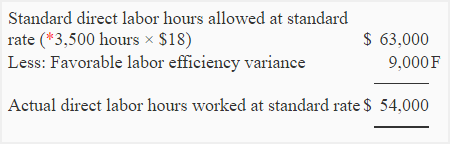Actual direct labor hours worked = Actual direct labor hours worked at standard rate/Standard rate per hour

= \$54,000/\$18

= 3,000 hours

*1,400 units × 2.5 hours

### (5) Direct labor rate variance:

(Actual hours worked × actual rate) – (Actual hours worked × standard rate)

= \$57,000 – (*3,000 hours × \$18)

= \$57,000 – \$54,000

= \$3,000 Unfavorable

*See answer to requirement 4

Show your love for us by sharing our contents.

### 2 Comments on Problem-4 (Materials and labor variances; computations from incomplete data)

1.Gabrielle

Yeah, that’s the titcke, sir or ma’am

2.James

When computing direct materials price variance, we need some data. How is this data collected? Help